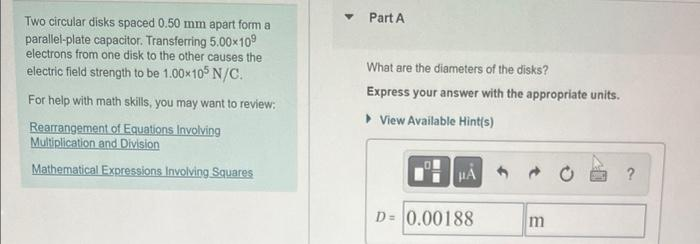# Question Two circular disks spaced 0.50 mm apart form a parallel-plate capacitor. Transferring 5.00×109 electrons from one disk to the other causes the electric field strength to be 1.00×105 N/C. For help with math skills, you may want to review: Rearrangement of Equations Involving Multiplication and Division Mathematical Expressions Involving Squares Part A What Two circular disks spaced 0.50 mm apart form a parallel-plate capacitor. Transferring 5.00×109 electrons from one disk to the other causes the electric field strength to be 1.00×105 N/C. For help with math skills, you may want to review: Rearrangement of Equations Involving Multiplication and Division Mathematical Expressions Involving Squares Part A What are the diameters of the disks? Express your answer with the appropriate units. ▸ View Available Hint(s) μÁ ? D= 0.00188 m